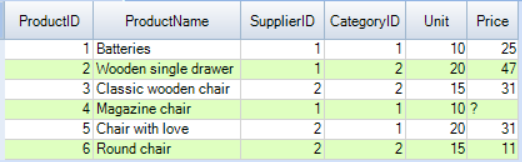Teradata SUM function returns the arithmetic sum of value_expression or specific column.

## Syntax

```SELECT SUM(DISTINCT/ALL value_expression )
FROM tables
WHERE predicates;```

Here,

• DISTINCTDISTINCT is used in the SUM function to eliminates all the duplicates and non-null while calculating the sum.
• ALL – This is the default value. In this case, all non-null values including duplicates will be counted while calculating the sum for a specific column or value_expression.
• value_expression – a literal or column expression for which the sum needs to be computed. The expression cannot contain any ordered analytical or aggregate functions.

## Examples

Let’s take an example to understand the concept of the Teradata SUM function.

### Table Preparation

Let’s create a volatile table name products and insert some dummy data into it.

```CREATE VOLATILE TABLE Products
(
ProductID INT NOT NULL,
ProductName varchar(100) NOT NULL,
SupplierID INT NOT NULL,
CategoryID INT NOT NULL,
Unit INT NOT NULL,
Price INT
)
unique primary index(ProductID)
on commit preserve rows;```
```insert into products (1,'Batteries',1,1,10,25);
insert into products (2,'Wooden single drawer',1,2,20,47);
insert into products (3,'Classic wooden chair',2,2,15,31);
insert into products (4,'Magazine chair',1,1,10,NULL);
insert into products (5,'Chair with love',2,1,20,31);
insert into products (6,'Round chair',2,2,15,11);```

Now you can check the data of the table using the select statement.

`select * from Products order by 1;`

OutputThe below statement will calculate the total number of units from the Products table.

`select sum(unit) as Total_Units from products;`

This is the same as,

`select sum(ALL unit) as Total_Units from products;`

Output

 Total_Units 90

`select sum(distinct unit) as Total_Units from products;`

Output

 Total_Units 45

You can see from the above output that the duplicate values have been ignored while calculating the sum.

### Teradata SUM with Group By

```select CategoryID, sum(Unit) as Total_Units from products
group by CategoryID;```

Output

 CategoryID Total_Units 1 40 2 50